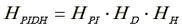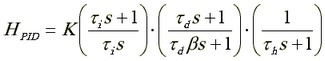﻿ 20-sim webhelp > Toolboxes > Control Toolbox > Filter Editor > Controllers > PID-Compensator

# PID-Compensator

The PID Compensator consists of an integrator and differentiator with proportional gain (PID Controller) together with a high-frequency roll-off filter.

 • Gain K: The controller gain K determines the frequency gain of the controller in the pass band (where integration and differentiation are of no interest).
 • Integration tau_i: The integration time constant tau i determines the frequency fi = 1 / tau_i [rad/s] after which the integrating behavior stops.
 • Differentiation tau_d: The differentiation time constant tau d determines the frequency fd = 1 / tau_d [rad/s] after which the differentiation behavior starts.
 • HF roll-off tau_h: The filter time constant tau h determines the frequency fh = 1 / tau_h [rad/s] of the high-frequency roll-off filter. Frequencies higher than fh are filtered out.
 • Tameness beta: The tameness factor beta influences the differentiating behavior. After frequency fdb = fd / beta the differentiation behavior stops. Decreasing beta increases this frequency fdb, resulting in a more pure differentiating behavior and therefore in a reduce of differentiation tameness.

## Transfer function of the controller:that equals